# Chemical Reactions 1 Chemical Reactions Chemical reactions or

• Slides: 31“Chemical Reactions” 1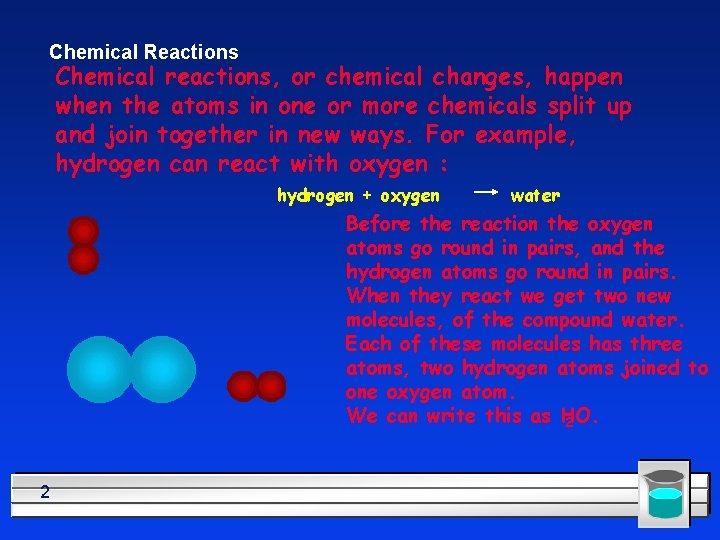Chemical Reactions Chemical reactions, or chemical changes, happen when the atoms in one or more chemicals split up and join together in new ways. For example, hydrogen can react with oxygen : hydrogen + oxygen water Before the reaction the oxygen atoms go round in pairs, and the hydrogen atoms go round in pairs. When they react we get two new molecules, of the compound water. Each of these molecules has three atoms, two hydrogen atoms joined to one oxygen atom. We can write this as H 2 O. 2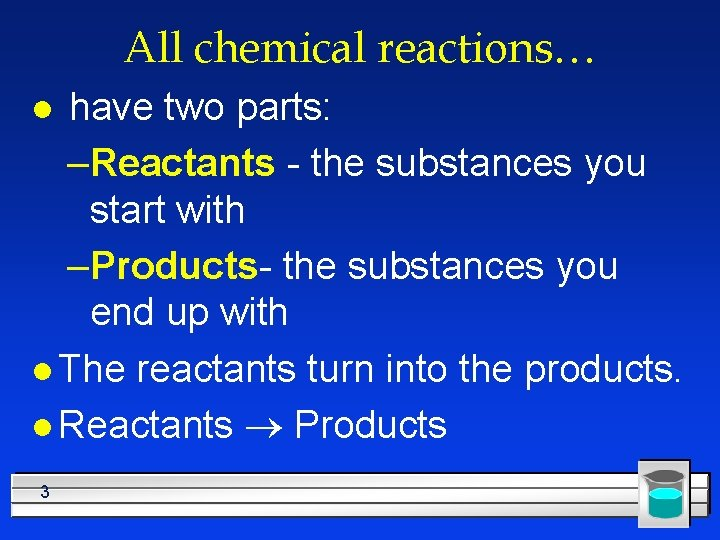All chemical reactions… have two parts: –Reactants - the substances you start with –Products- the substances you end up with l The reactants turn into the products. l Reactants ® Products l 3Chemical Changes Products Reactants 4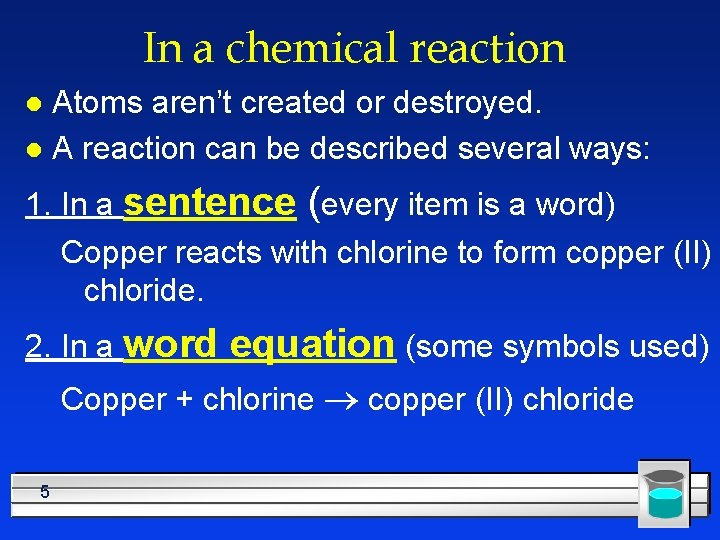In a chemical reaction Atoms aren’t created or destroyed. l A reaction can be described several ways: l 1. In a sentence (every item is a word) Copper reacts with chlorine to form copper (II) chloride. 2. In a word equation (some symbols used) Copper + chlorine ® copper (II) chloride 5Symbols in equations the arrow separates the reactants from the products – Read as “reacts to form” or yields l The plus sign = “and” l (s) after the formula = solid: Ag. Cl(s) l (g) after the formula = gas: CO 2(g) l (l) after the formula = liquid: H 2 O(l) l 6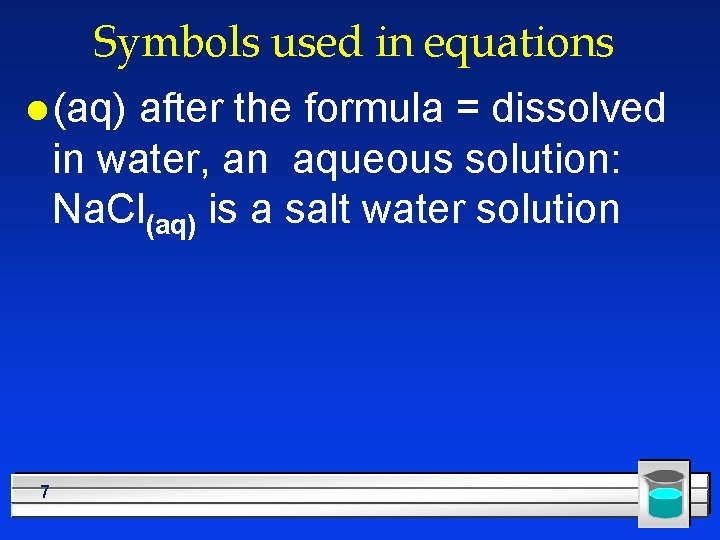Symbols used in equations l (aq) after the formula = dissolved in water, an aqueous solution: Na. Cl(aq) is a salt water solution 7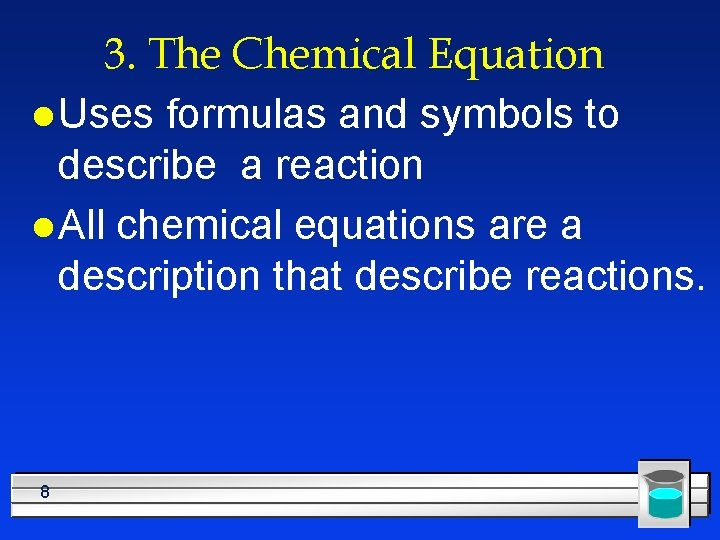3. The Chemical Equation l Uses formulas and symbols to describe a reaction l All chemical equations are a description that describe reactions. 8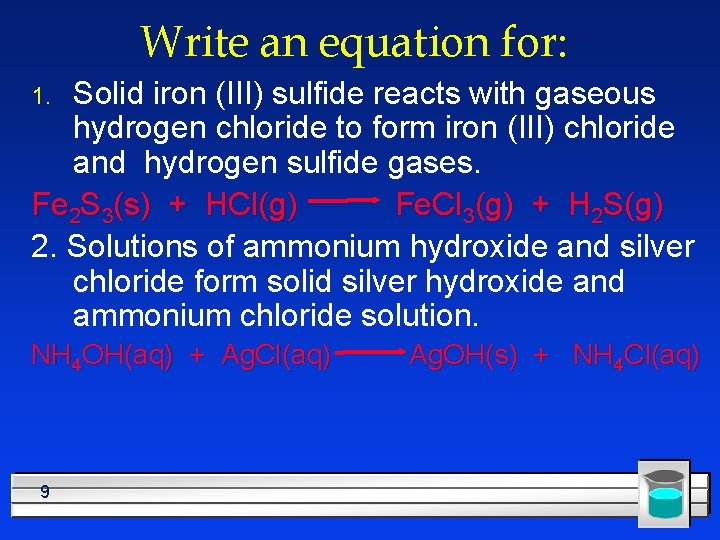Write an equation for: Solid iron (III) sulfide reacts with gaseous hydrogen chloride to form iron (III) chloride and hydrogen sulfide gases. Fe 2 S 3(s) + HCl(g) Fe. Cl 3(g) + H 2 S(g) 2. Solutions of ammonium hydroxide and silver chloride form solid silver hydroxide and ammonium chloride solution. 1. NH 4 OH(aq) + Ag. Cl(aq) 9 Ag. OH(s) + NH 4 Cl(aq)Now, read these: Fe(s) + O 2(g) ® Fe 2 O 3(s) Cu(s) + Ag. NO 3(aq) ® Ag(s) + Cu(NO 3)2(aq) NO 2 (g) 10 N 2(g) + O 2(g)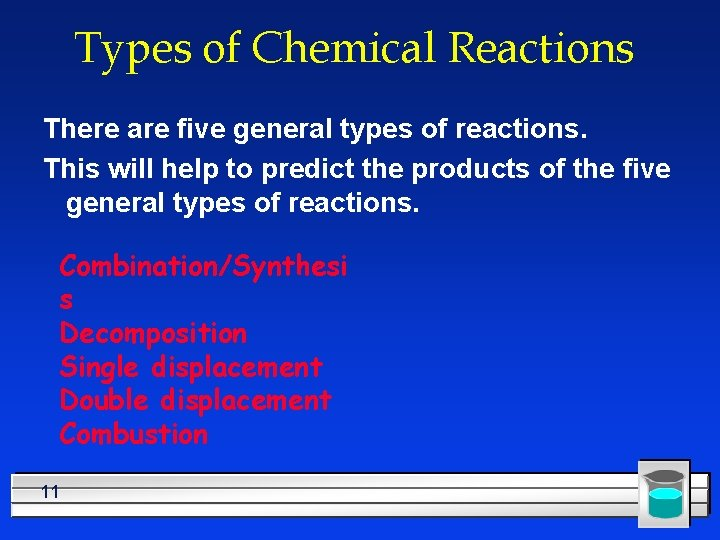Types of Chemical Reactions There are five general types of reactions. This will help to predict the products of the five general types of reactions. Combination/Synthesi s Decomposition Single displacement Double displacement Combustion 11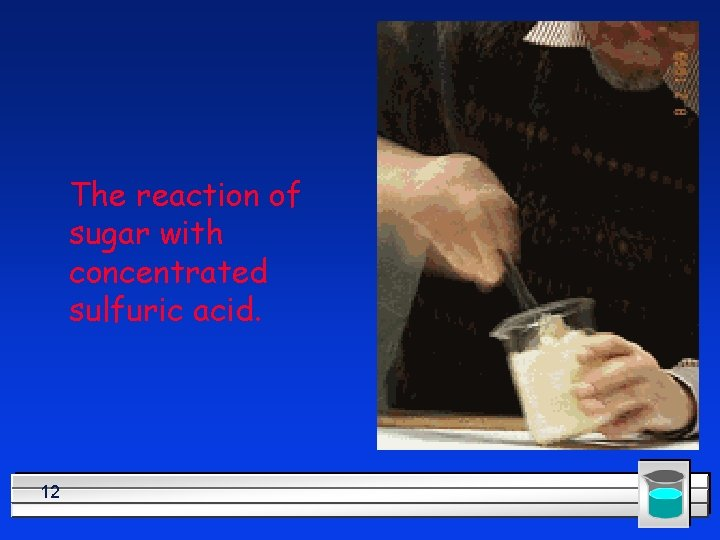The reaction of sugar with concentrated sulfuric acid. 12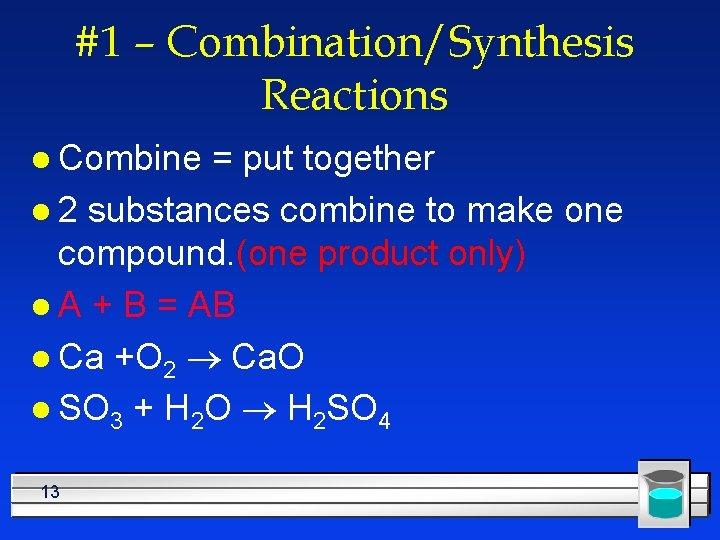#1 – Combination/Synthesis Reactions l Combine = put together l 2 substances combine to make one compound. (one product only) l A + B = AB l Ca +O 2 ® Ca. O l SO 3 + H 2 O ® H 2 SO 4 13+ 14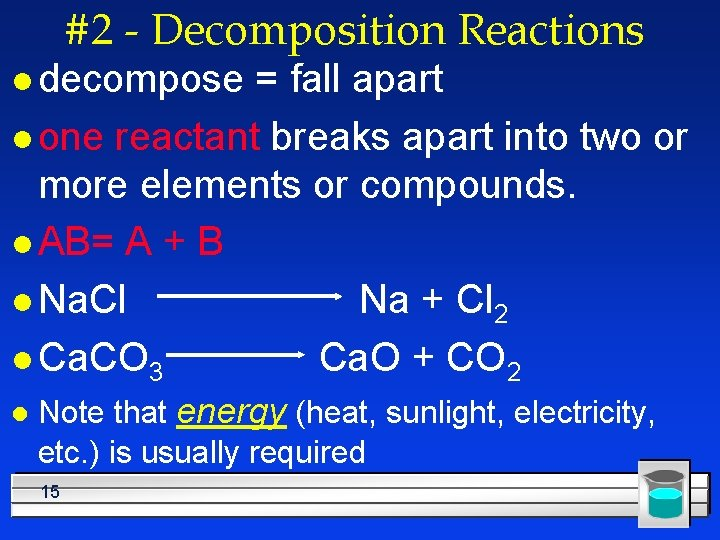#2 - Decomposition Reactions l decompose = fall apart l one reactant breaks apart into two or more elements or compounds. l AB= A + B l Na. Cl Na + Cl 2 l Ca. CO 3 Ca. O + CO 2 l Note that energy (heat, sunlight, electricity, etc. ) is usually required 15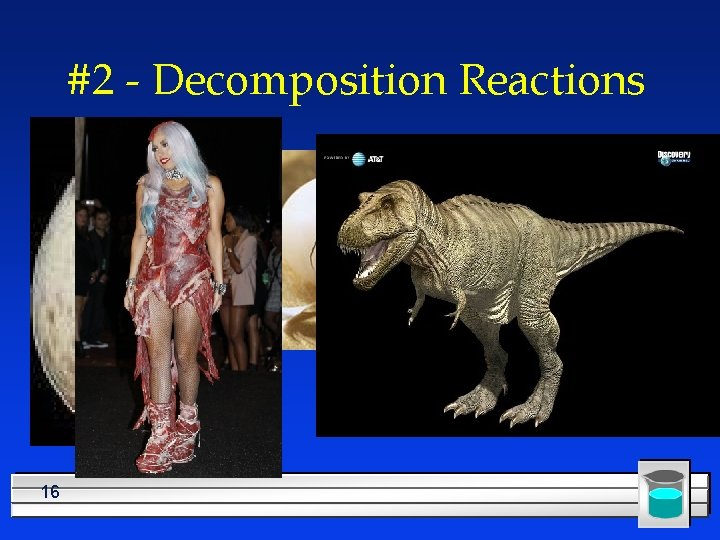#2 - Decomposition Reactions 1617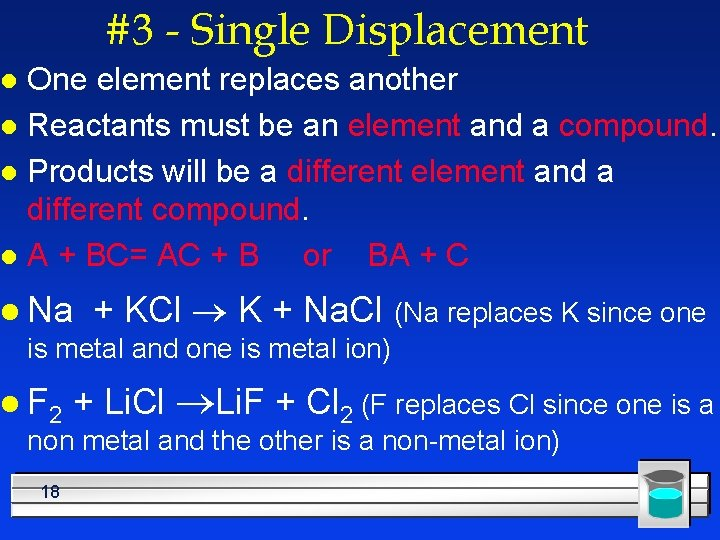#3 - Single Displacement One element replaces another l Reactants must be an element and a compound. l Products will be a different element and a different compound. l A + BC= AC + B or BA + C l l Na + KCl ® K + Na. Cl (Na replaces K since one is metal and one is metal ion) l F 2 + Li. Cl ®Li. F + Cl 2 (F replaces Cl since one is a non metal and the other is a non-metal ion) 18#3 - Single Displacement + 19+ 20#3 Single Displacement Practice: l Fe + Cu. SO 4 ® l Pb + KCl ® l Al + HCl ® 21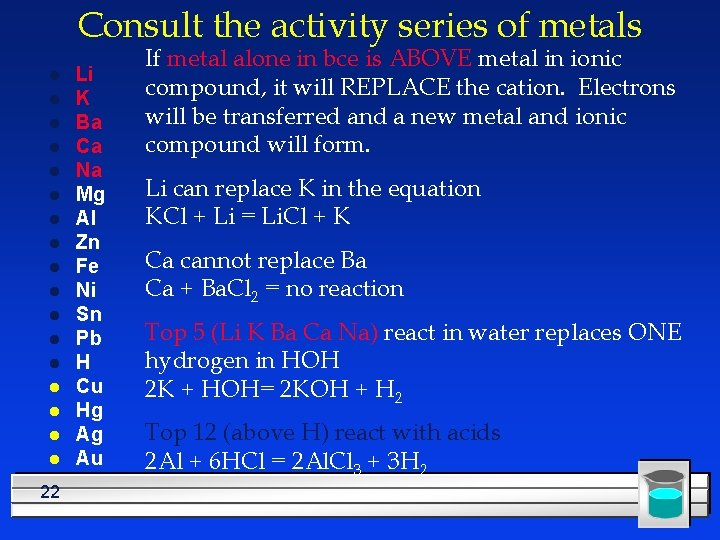Consult the activity series of metals l l l l l 22 Li K Ba Ca Na Mg Al Zn Fe Ni Sn Pb H Cu Hg Ag Au If metal alone in bce is ABOVE metal in ionic compound, it will REPLACE the cation. Electrons will be transferred and a new metal and ionic compound will form. Li can replace K in the equation KCl + Li = Li. Cl + K Ca cannot replace Ba Ca + Ba. Cl 2 = no reaction Top 5 (Li K Ba Ca Na) react in water replaces ONE hydrogen in HOH 2 K + HOH= 2 KOH + H 2 Top 12 (above H) react with acids 2 Al + 6 HCl = 2 Al. Cl 3 + 3 H 2Consult the activity series for non-metals F l Cl l Br l. I l If non metal alone in bce is ABOVE non -metal in ionic compound, it will REPLACE the anion. Electrons will be transferred and a new metal and ionic compound will form. F can replace Cl in the equation 2 KCl + F 2 = 2 KF + Cl 2 Br cannot replace Cl Br 2 + Ba. Cl 2 = no reaction 23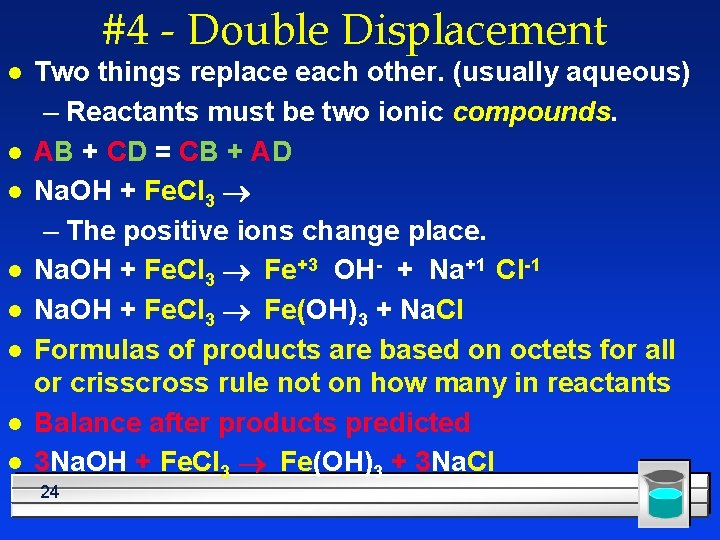#4 - Double Displacement l l l l Two things replace each other. (usually aqueous) – Reactants must be two ionic compounds. AB + CD = CB + AD Na. OH + Fe. Cl 3 ® – The positive ions change place. Na. OH + Fe. Cl 3 ® Fe+3 OH- + Na+1 Cl-1 Na. OH + Fe. Cl 3 ® Fe(OH)3 + Na. Cl Formulas of products are based on octets for all or crisscross rule not on how many in reactants Balance after products predicted 3 Na. OH + Fe. Cl 3 ® Fe(OH)3 + 3 Na. Cl 24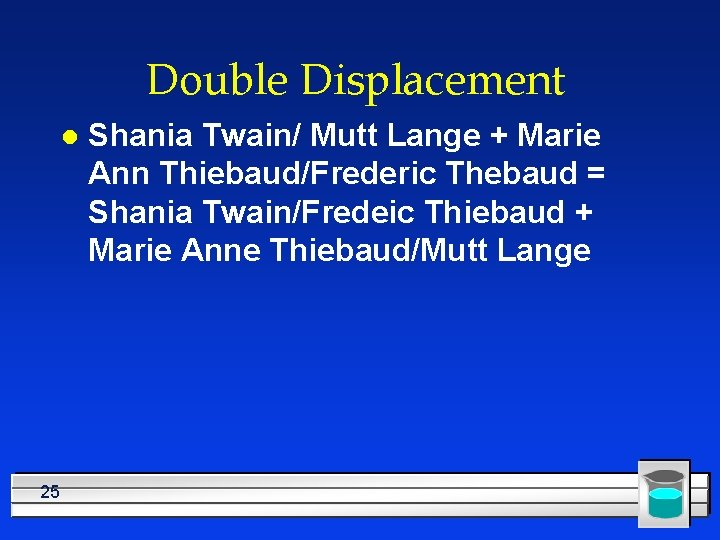Double Displacement l 25 Shania Twain/ Mutt Lange + Marie Ann Thiebaud/Frederic Thebaud = Shania Twain/Fredeic Thiebaud + Marie Anne Thiebaud/Mutt Lange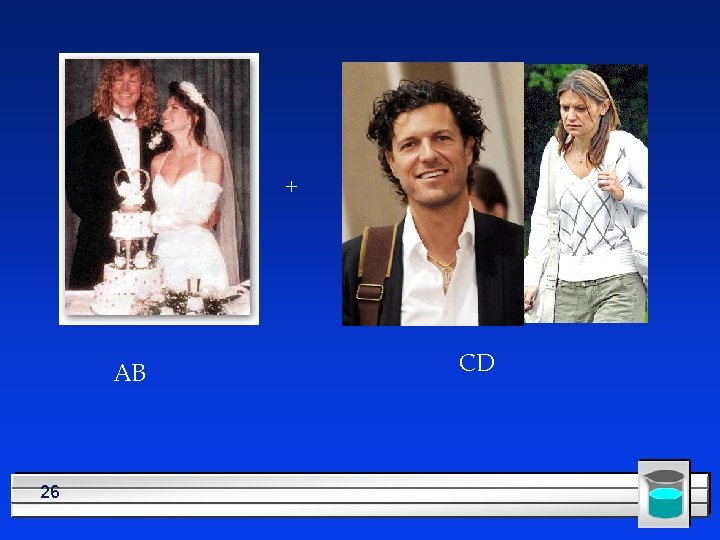+ AB 26 CD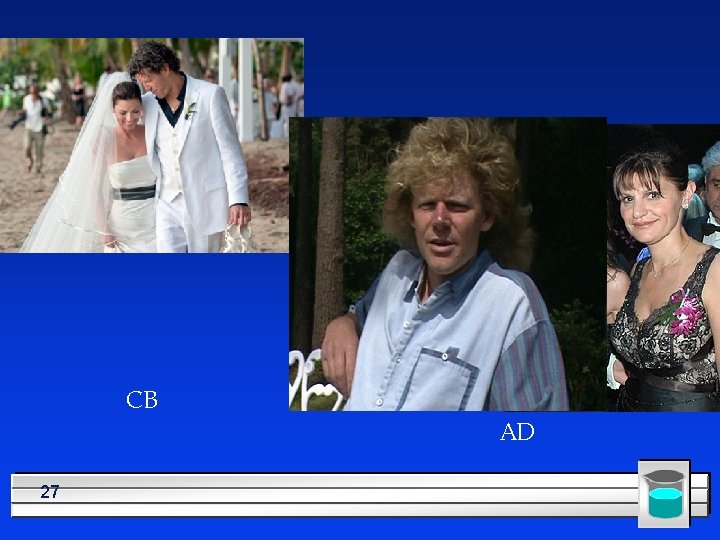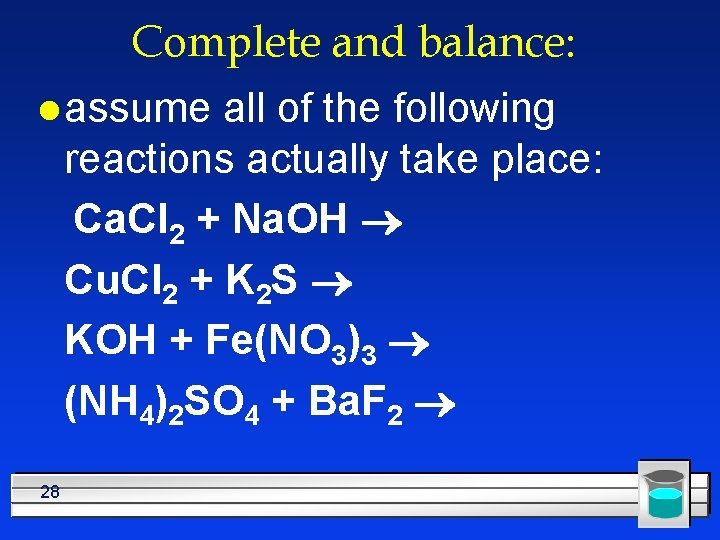Complete and balance: l assume all of the following reactions actually take place: Ca. Cl 2 + Na. OH ® Cu. Cl 2 + K 2 S ® KOH + Fe(NO 3)3 ® (NH 4)2 SO 4 + Ba. F 2 ® 28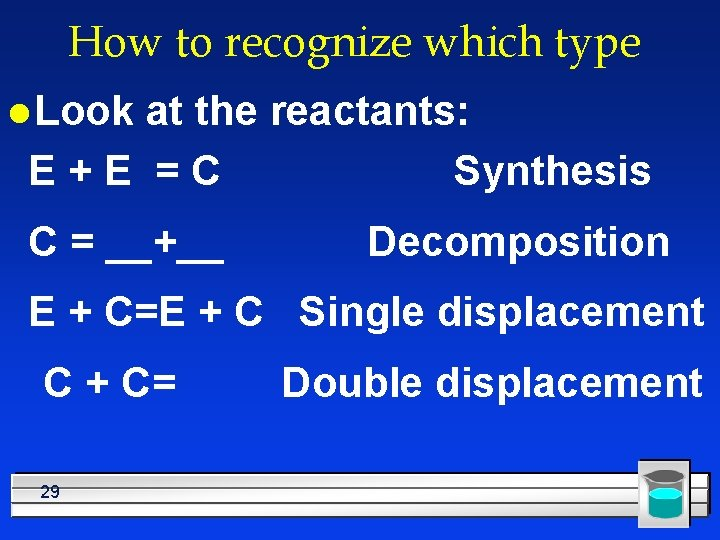How to recognize which type l Look at the reactants: E+E =C Synthesis C = __+__ Decomposition E + C=E + C Single displacement C + C= 29 Double displacement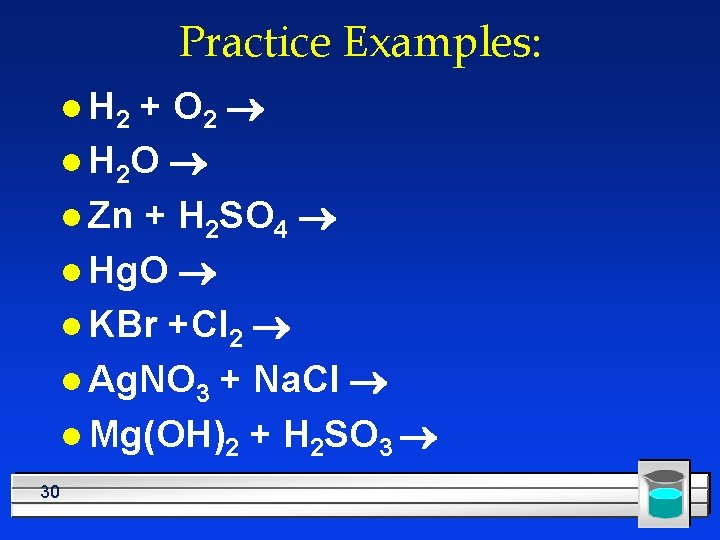Practice Examples: + O 2 ® l H 2 O ® l Zn + H 2 SO 4 ® l Hg. O ® l KBr +Cl 2 ® l Ag. NO 3 + Na. Cl ® l Mg(OH)2 + H 2 SO 3 ® l H 2 30#5 - Combustion Means “add oxygen” l Normally, a compound composed of only C, H, (and maybe O) is reacted with oxygen – usually called “burning” l If the combustion is complete, the products will be CO 2 and H 2 O water. l If the combustion is incomplete, the products will be CO (or possibly just C) and H 2 O water. l Cx. Hy + O 2 = CO 2 + H 20 l C 8 H 18 + 12. 5 O 2 = 8 CO 2 + 9 H 20 l. C+E=C+C l 31